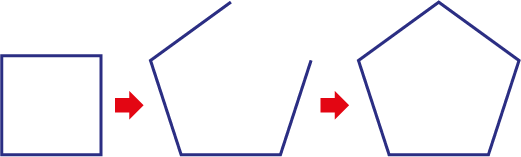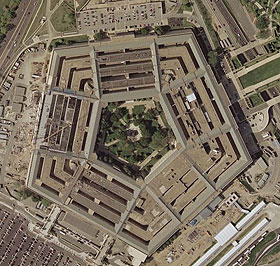# Growing polygons

Here is a fun patterning activity for introducing the names of some polygons.

Start by considering only polygons that have equal sides and equal angles.

Make sure that students know the names of a triangle and a square. Then ask the question:
How can you make the triangle into a square?A triangle and a square.

Students should see that they need to open up the triangle and add another side of the same length.Making a triangle into a square.

Continue. Open up the square and add another side. What do you get now? Some students may know that this shape is called a pentagon.Making a square into a pentagon.The Pentagon in Washington DC, USA.

Continue to hexagon, heptagon, octagon… as far as students want to go. Make a chart summarising the names and the number of sides.

Now inform students that the polygons they have been drawing are called regular polygons.

Ask students to add some examples of irregular polygons to their chart.

Stress that polygons with the same number of sides have the same name whether they are regular or not.